# 4.1 The Basic Structure

Conceptually, a skiplist is a sequence of singly-linked lists. Each listcontains a subset of the items in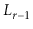. We start with the input list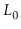that contains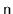items and constructfrom,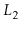from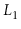, and so on. The items in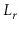are obtained by tossing a coin for each element,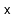, inand includinginif the coin turns up as heads. This process ends when we create a listthat is empty. An example of a skiplist is shown in Figure 4.1.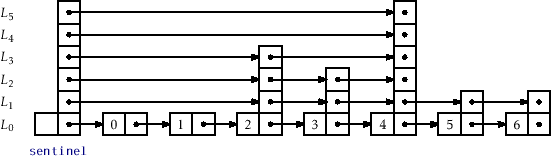For an element,, in a skiplist, we call the height ofthe largest value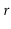such thatappears in. Thus, for example, elements that only appear inhave height 0. If we spend a few moments thinking about it, we notice that the height ofcorresponds to the following experiment: Toss a coin repeatedly until it comes up as tails. How many times did it come up as heads? The answer, not surprisingly, is that the expected height of a node is 1. (We expect to toss the coin twice before getting tails, but we don't count the last toss.) The height of a skiplist is the height of its tallest node.

At the head of every list is a special node, called the sentinel, that acts as a dummy node for the list. The key property of skiplists is that there is a short path, called the search path, from the sentinel in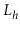to every node in. Remembering how to construct a search path for a node,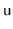, is easy (see Figure 4.2) : Start at the top left corner of your skiplist (the sentinel in) and always go right unless that would overshoot, in which case you should take a step down into the list below.

More precisely, to construct the search path for the nodein, we start at the sentinel,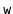, in. Next, we examine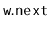. Ifcontains an item that appears beforein, then we set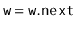. Otherwise, we move down and continue the search at the occurrence ofin the list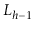. We continue this way until we reach the predecessor ofin.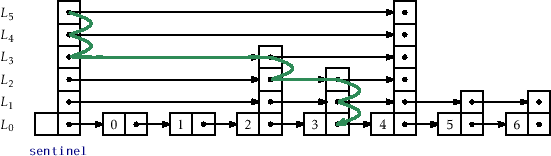The following result, which we will prove in Section 4.4, shows that the search path is quite short:

Lemma 4..1   The expected length of the search path for any node,, inis at most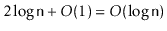.

A space-efficient way to implement a skiplist is to define a Node,, as consisting of a data value,, and an array,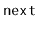, of pointers, where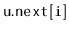points to's successor in the list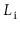. In this way, the data,, in a node is referenced only once, even thoughmay appear in several lists.

```    class Node<T> {
T x;
Node<T>[] next;
Node(T ix, int h) {
x = ix;
next = (Node<T>[])Array.newInstance(Node.class, h+1);
}
int height() {
return next.length - 1;
}
}
```

The next two sections of this chapter discuss two different applications of skiplists. In each of these applications,stores the main structure (a list of elements or a sorted set of elements). The primary difference between these structures is in how a search path is navigated; in particular, they differ in how they decide if a search path should go down intoor go right within.

opendatastructures.org